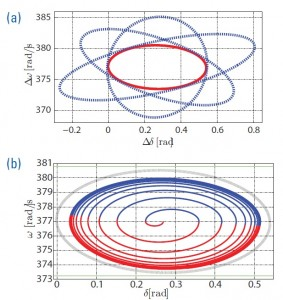Christine Chen with adviser A. DomÃ­nguez-GarcÃ­a

The efforts to reduce our dependence on fossil fuels as a major source of electrical energy requires integrating renewable resources into the power grid. As these are generally highly variable, new tools are necessary to assess their impact on power system operational security. We propose using ellipsoidal methods. Our interest is in how system variables, such as frequency and bus angles, deviate from values imposed by operational requirements due to wind variability.
We assume that power system dynamics are described by the classical nonlinear differential-algebraic equation (DAE) representation, where the effect of the wind resource is modeled as an uncontrolled disturbance to the system dynamics. Moreover, we assume the disturbance introduced by wind variability is small enough to justify the use of a small-signal model. Then, the DAE model is linearized around some nominal trajectory determined by the wind forecast. The forecast errors provide bounds on the variability of wind-based generation injected into the system. These bounds are used together with the linearized model to compute the reach set, which provides bounds on worst-case deviations of system variables of interest, e.g., frequency and voltage at certain buses.Figure 18: (a) Tight ellipsoidal bounds of the linearized model reach set and reach set obtained as the intersection of the tight ellipsoidal family. (b) Large-signal worse-case trajectory and linearized model reachability set.

A simple illustrative example is the single-machine infnite bus system as shown in Figure 17 where the input wind variability is embedded in vâˆž. Output variables of interest are the frequency (Ï‰) and angle (Î´) of the machine. In Figure 18(a), a few ellipsoids of the ellipsoidal family are represented in dashed traces, the intersection of which yields the linearized system reach set. In the same figure, the reach set is depicted in the solid trace. The linearized model reach set is reproduced in Figure 18(b) as the dashed line. For comparison, the worst-case system trajectory of the nonlinear DAE is plotted in the same figure. This indicates the linearized model is, indeed, a close approximation of the nonlinear system as long as the input variability is within a tolerable range.
We are currently working on extending these ideas to large-scale systems with the aid of Power System Toolbox in conjunction with our custom MATLAB code.

This research is partially supported by the National Science Foundation under grant.